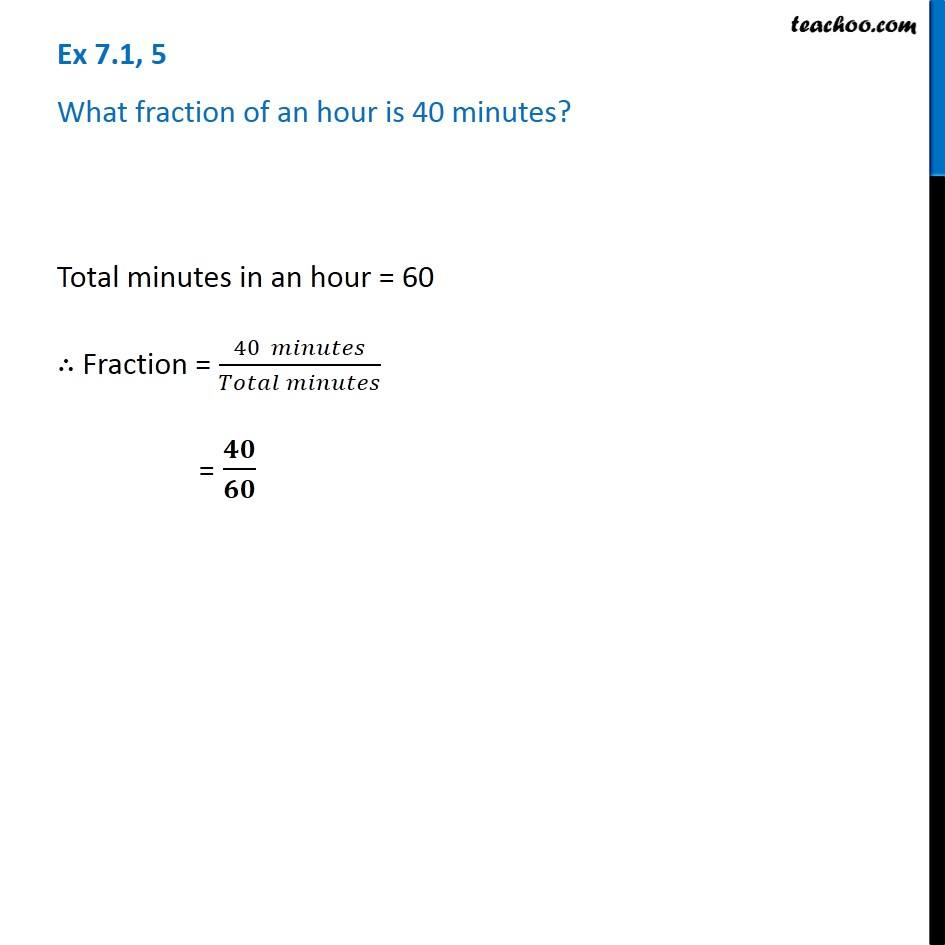1. Chapter 7 Class 6 Fractions
2. Concept wise
3. Finding fraction

Transcript

Ex 7.1, 5 - Chapter 7 Class 6 NCERT - Fractions What fraction of an hour is 40 minutes? Total minutes in an hour = 60 ∴ Fraction = (40 minutes) / (Total Minutes) = 40/60